Prediction Method of Maximum Seismic Response for Vibration Control Steel Frames With Scaling-Frame Device Installed | OMICS International
Journal of Civil & Environmental Engineering

# Prediction Method of Maximum Seismic Response for Vibration Control Steel Frames With Scaling-Frame Device Installed

Akinobu Kano1*, Hideaki Azu1, Takumi Ito1, Donghung Wu2, Takashi Nagumo3 and Haruhiko Hirata3

1Department of Architechture, Tokyo University of Science, Japan

2Wu Office, P.R. Japan

3Hory Corporation, Japan

*Corresponding Author:
Akinobu Kano
Department of Architechture
Tokyo University of Science, Japan
Tel: +81(0)3-5228-8726
E-mail: [email protected]

Received date: July 06, 2016; Accepted date: August 18, 2016; Published date: August 20, 2016

Citation: Kano A, Azu H, Ito T, Wu D, Nagumo T, et al. (2016) Prediction Method of Maximum Seismic Response for Vibration Control Steel Frames With Scaling-Frame Device Installed. J Civil Environ Eng S3:007. doi:10.4172/2165-784X.S3-007

Copyright: © 2016 Kano A, et al. This is an open-access article distributed under the terms of the Creative Commons Attribution License, which permits unrestricted use, distribution, and reproduction in any medium, provided the original author and source are credited.

Visit for more related articles at Journal of Civil & Environmental Engineering

#### Abstract

The innovated vibration control device called as scaling frame à²¯SFà²° structure has been proposed by the authors, and the SF structures are applied to low-rise wooden structures already. This paper aims to investigate the applicability of SF structure on steel frames. Herein, SF structure consists of beam-column frame, diagonal bracing, and SF device à²¯SFDà²° installed, and SFD is made of Steel or aluminum. And vibration energy is absorbed by the plastic behavior of the diagonal deformation of SF device. In previous study, the experimental study on SFD and steel frame specimen with SFD installed were conducted to clarify the seismic response and seismic mitigation effect. And also, from observation of test results, analytical method has been suggested. From the comparison of test results, the proposed method shows good agreements with test results. Furthermore, the enormous past studies have suggested prediction method of maximum seismic response of vibration control frames. So this study aim to develop the prediction method of seismic response on vibration controlled steel frames with SFD installed, and the simple design procedure can be provided. The first, the simplified restoring force characteristics which can chase the test results is applied on prediction method procedure. So the prediction method is reformulated to adapt the bilinear model and strain hardening rule. The time history response analysis is performed to investigate the applicability and effectiveness. From the comparison of analysis results and predictions, it is confirmed that the proposed prediction method shows enough accuracy.

#### Keywords

Prediction method of maximum response; Steel framework; Seismic vibration control system; Time history response analysis

#### Introduction

Recently, a various type of vibration control device and damper has been developed on country, and most devices are suggested and adopted to actual building structure. For example, oil damper and high damping rubber device are suggested. However, they have problems of durability and stability velocity. In addition, they consist of special device and material, so there are some difficulties of getting material and construction technics. In contrast, the innovated vibration control device called as scaling frame (abbreviated as “SF”) structure has been proposed by the authors, and it is expected that SF structure can solve these difficulties. SF structure consists of beam-column frame, diagonal bracing, and SF device (abbreviated as “SFD”) installed. SF as shown in Figure 1. From the points of mechanical characteristics and materials, SFD are categorized as shown Table 1 with damping system, resistant mechanics and materials. SFD is made of Steel or aluminum. And vibration energy is absorbed by the plastic behavior of the diagonal deformation of SFD. SFD has some advantage such as workability, transportability and containment because of small size, light weight, durability and sustainability of metal materials. And SF structures are applied to low-rise wooden structures already. This paper aims to investigate the applicability of SF structure on steel frames. (Figure 2) This study aim to develop the prediction method of seismic response on vibration controlled steel frames with SFD installed, and the simple design procedure can be provided. To investigate the simplified restoring force characteristics, the horizontal static cyclic loading test is conducted on half-scaled frame test [1,2]. And, the prediction method is reformulated to adapt the bilinear model and strain hardening rule. To investigate the applicability and effectiveness of the method, the time history response analysis is performed geometrically. From Figure 3, it can be said that the compressive displacement grows larger than the diagonal displacement of extension. Thus, the diagonal member on tensile side will resist increasingly, on the other hands, the diagonal member on compressive side will be reduced as shown in Figure 4. So the lateral buckling of diagonal member on compressive side is prevented, and bending reflection of the SF is restricted in large deformation range, and rigidity in the axial direction of the SFD becomes dominant (Figure 5).

Mechanics System Materials
Bending Viscous Metal
Shear Hysteresis Oil
Axial Friction Rubber

Table 1: The mechanical characteristics and materials of SFD.

General Description of SF Structure

Herein, the general description of resistant mechanism and characteristics of SF structure is explained. As a definition of SF structure, the relation of size of out-frames and SFD is defined as reduction rate α as shown in Figure 1. Figure 2 shows the resistant mechanism of SF structure. From the principal of vertical work, the rigidity KSF and yield strength Py are obtained as follows: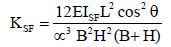(1)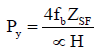(2)

E: The young’s modulus of the SFD

ISF: Moment of inertia of area of the SFD

ZSF: Elastic modulus of SFD

B: The length of the beam

H: The length of the column

L: The diagonal length of beam-column frame

fb: Allowable bending stress of the SFD

From the above equations, it can be predicted that KSF is inverse proportion to a cube of reduction rate α, and the strength is inverse proportion to reduction rate α. Therefore, SF structure with small reduction ratio shows the large rigidity, strength and high energy absorption. However, in case of very small reduction rate of SFD, the fracture would be occurred, so it is desirable that the limitation of reduction ratio is decided. The next, the tensile and compressive deformation in the diagonal displacement of SFD subjected to lateral force are dominated, it means that the diagonal bracing system is appeared. So the tensile and compressive displacements of each member are drawn in Figure 3 geometrically. From Figure 3, it can be said that the compressive displacement grows larger than the diagonal displacement of extension. Thus, the diagonal member on tensile side will resist increasingly, on the other hands, the diagonal member on compressive side will be reduced as shown in Figure 4. So the lateral buckling of diagonal member on compressive side is prevented, and bending reflection of the SF is restricted in large deformation range, and rigidity in the axial direction of the SFD becomes dominant.

This paper investigates the fundamental restoring force characteristics of SFD experimentally. Herein, the horizontal static loading tests are conducted on elementally test to investigate them. Figure 5 shows the test specimen of SFD. Herein, the reduction ratio of SFD is considered with parameters as 7%, 8.5%, and 10%. SFD (thickness 19 mm) are made of steel. Table 2 shows member of test specimen and test parameters on elementally test and the mechanical properties of steel used for the test specimen. Herein, α is reduction rate. σy is yield strength and σu is tensile strength. And, E is Young’s modulus. SS400 present the grade of JIS (Japanese Industrial Standards). This paper investigates the fundamental restoring force characteristics of SFD experimentally. Herein, the horizontal static loading tests are conducted on elementally test to investigate them. Figure 5 shows the test specimen of SFD. Herein, the reduction ratio of SFD is considered with parameters as 7%, 8.5%, and 10%. SFD (thickness 19 mm) are made of steel. Table 2 shows member of test specimen and test parameters on From the above equations, it can be predicted that KSF is inverse proportion to a cube of reduction rate α, and the strength is inverse proportion to reduction rate α. Therefore, SF structure with small reduction ratio shows the large rigidity, strength and high energy absorption. However, in case of very small reduction rate of SFD, the fracture would be occurred, so it is desirable that the limitation of reduction ratio is decided. The next, the tensile and compressive deformation in the diagonal displacement of SFD subjected to lateral force are dominated, it means that the diagonal bracing system is appeared. So the tensile and compressive displacements of each member are drawn in Figure 3 elementally test and the mechanical properties of steel used for the test specimen. Herein, α is reduction rate. σy is yield strength and σu is tensile strength. And, E is Young’s modulus. SS400 present the grade of JIS (Japanese Industrial Standards) [3,4].

Member Reductionratio JISgrade σy(N/mm2) σu(N/mm2) E(kN/mm2)
S-α-7 7 SS400   285   433   210
S-α-8.5 8.5 SS400
S-α-10 10 SS400
Bracing - SS400 342 472 202

Table 2: Name of test specimen and test parameters and mechanical properties of steel.

Figure 6 shows a loading test setup. In this paper, the length of column is 1800 mm, and that of beam is 1000 mm on half-scaled frame. SFD connected with column jig through gusset plate and diagonal bracing. Columns are supported to the beam by pin joint.

The horizontal force is measured from the load cell built into the loading jack. The horizontal displacement at the top of the column is measured by using a tape measure type displacement transducer. The strain gauges are placed on the SFD and the diagonal bracing as shown in Figure 7. Loading pattern is monotonic or cyclic. The loading program is arranged by the reference of the target displacement, and the maximum angles of the column at each loop are 1/200, 1/100, 1/75, 1/50, and 1/30 rad. It is gradually increased and the same two angles of the column for each cycle are repeated (Table 3).

No Input Wave PGA(gal) Duration (s) Dh
1 El Centro NS 341.7 53.76 25
2 Hachinohe NS 229.65 50.98 25
3 Taft NS 152.7 54.38 30
4 Fukiai NS 804.58 30 15
5 Tottori NS 714 60 25
6 Miyagi NS 1093.66 60 25
7 Niigata NS 863.06 60 15
8 Takatori EW 605.55 60 20
9 JMA-Kobe NS 818 39.33 15
10 BCJ-L2 355.66 120 75
11 Ocean EQ(GPV100kine) 196.55 81.93 75
12 Epicentral EQ(GPV100kine) 671.15 81.93 7

Table 3: List of input seismic wave.

#### Result of the Test Study

Figure 8 shows the relation of horizontal load - horizontal displacement of monotonic loading test result. From the test results of Figure 8, it is confirmed that the rigidity and strength of the SFD become large in case of small reduction rate α. And also, the strain hardening is appeared during ultimate state. Moreover, strength and rigidity of SFD is raised strongly during ultimate states. From the analysis of axial and curvature of SFD obtained from strain gauges, the curvature is dominated during early stage, however the axial deformation is advanced during ultimate states. According to the above results, it can be said that resistance state of the SFD translates from the bending mechanism to axial force mechanism with progress of the deformation [1,2].

Figure 9 shows the relation of horizontal load - horizontal displacement of monotonic loading test result. From the results of Figure 9, it is confirmed that the stable inelastic behaviors are presented.

#### Proposal of Restoring Force Characteristics

From the test results of Figure 9, the skeleton curve of SFD is modeled as bi-linear curve as shown in Figure 10. A break point of bilinear curve is determined by the yield strength and the rigidity of SFD. It is the theoretical value based on Eq.(1),(2). And the second rigidity is determined from monotonic loading test of SFD and material testing. The ratio of initial rigidity and second rigidity are 0.04 in the steel SFD and 0.015 in the aluminum SFD. Herein, the hysteresis behavior model is assumed with the strain hardening model as shown in Figure 11. It’s confirmed that this model is the static state by several cycles after it passed through hardening phenomena repeatedly when repetitive stress was given under the constant distorted amplitude. In this paper, the analytical coefficient β related to hardening rule is decided to correspond with test result of SFD (herein, β=0.5). When β is 0.5; this model is the static state by two cycles. Herein, the hysteresis behavior model is the elasto-plastic force-displacement relation model, when the ratio of initial rigidity and second rigidity are 0. Figure 12 shows the comparison of horizontal load - horizontal displacement curves of the cyclic loading test and analytical results. Figure 13 shows the comparison of horizontal load - cumulative displacement curves of the cyclic loading test and analytical results. From the results of Figures 12 and 13, the analysis results can chase test results well.

#### Prediction Method of Maximum Seismic Response Based on Equivalent Linearization

In this paper, the strain hardening model of hysteresis rule is assumed as shown in Figure 14. The revised equivalent natural period Teq of vibration controlled frame is as follow: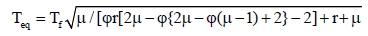(3)

Where, Tf is natural period of steel frame, r is ratio of elastic stiffness ( = kd/kf), kd is elastic stiffness of SFD, kf is elastic stiffness of frame, kb is elastic stiffness of bracing member, φ is second rigidity ratio of SFD, μ is maximum ductility. Then, the equivalent natural period of φ = 0 is as follow: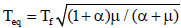(4)

And the hysteresis loop presents the perfectly elasto-plastic. Furthermore, by use of Newmark-Rosenblueth method , the equivalent damping heq is as follows: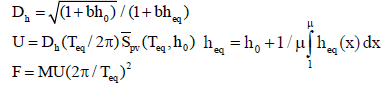(5)

Herein, larger maximum ductility μ leads to larger Teq and to a certain extent, larger heq.

Peak response of SFD will be obtained from common linear response spectrum as well as Teq and heq explained above. Ductility by each cycle is various and the period by each cycle change between T0 and Teq under seismic response included a various volume of amplitude. Then, maximum velocity by use average of velocity response spectrum from T0 to Teq is as follow: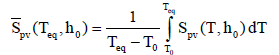(6)

The effect of damping increase is treated by the Dh. Dh represents the average reduction Sd, Spv and Spa for an ensemble of earthquakes over the entire range of vibration periods. It is given as follow :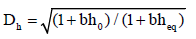(7)

The coefficient b is related to earthquake as shown in Table 3. Moreover, pseudo-displacement response spectrum and pseudoacceleration response spectrum is as follow: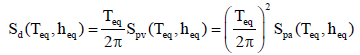(8)

Equivalent acceleration response spectrum is calculated by smoothing by an integration average of response spectrum and the extension of the period to maximum ductility μ as shown in Figures 15 and 16 (arrow (1)). Velocity response spectrum is predicted using the response reduction rate by the response is reduced by increase of damping by the extension of the period (arrow (2)). Therefore, maximum response displacement and maximum shear force are following as: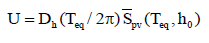(9)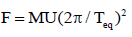(10)

#### Verification of Accuracy of Prediction method

Analytical frame model

At first, a single-story model is adopted as fundamental study as shown in Figure 17. The analytical frame model is pinned support at column base, and it is assumed that a column (H-300 × 300 × 10 × 15, SS400) and a beam (H-300 × 150 × 6.5 × 9) behave within elastic, so then SFD show the inelastic behavior. It is assumed that the inertial mass is 9000 kg and damping factor is 2% of the frame. Full plastic moment of the column is larger than that of the beam. A natural period of framework is determined from the inertial mass and initial stiffness of the whole framework. The P-δ Effect is not considered herein. In addition, Figure 18 shows the skeleton curve of whole frame.

Seismic response analysis

The time history response analysis is performed to investigate the applicability and effectiveness. The seismic response analysis is performed subjected to 12 waves which include the past records during earthquake disaster and artificial seismic waves. The damping effect coefficients Dh are summarized on Table 3. Ratio of elastic stiffness r is considered with parameters as 1, 4 and 9. Yield deformation ration y is considered with parameters as 3, 4, 5 and 6.

#### The Comparison of Analysis, Results and Predictions

Figure 19 shows the comparison of Prediction values by use prediction method and results of the time history response analysis. Figure 19 shows the comparison of maximum response displacement and maximum shear force. Furthermore, the mean value and the standard deviation of result of comparison are shown the same figure. From the comparison of analysis results and predictions, it is confirmed that the proposed prediction method shows enough accuracy. Moreover, it is confirmed that damping evaluation show approximate accuracy when the hysteresis behavior model is assumed with the strain hardening model.

#### Conclusions

1) The prediction method is reformulated to adapt the bilinear model and strain hardening rule.

2) Prediction method of maximum seismic response based on equivalent linearization technique shows enough accuracy.

#### References

Select your language of interest to view the total content in your interested language

### Article Usage

• Total views: 8974
• [From(publication date):
specialissue-2016 - Jan 28, 2020]
• Breakdown by view type
• HTML page views : 8887Can't read the image? click here to refresh